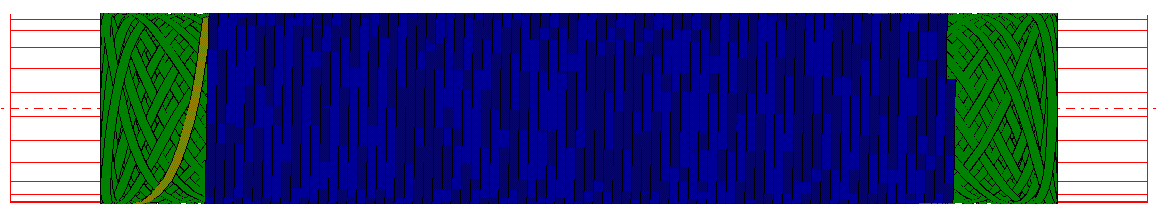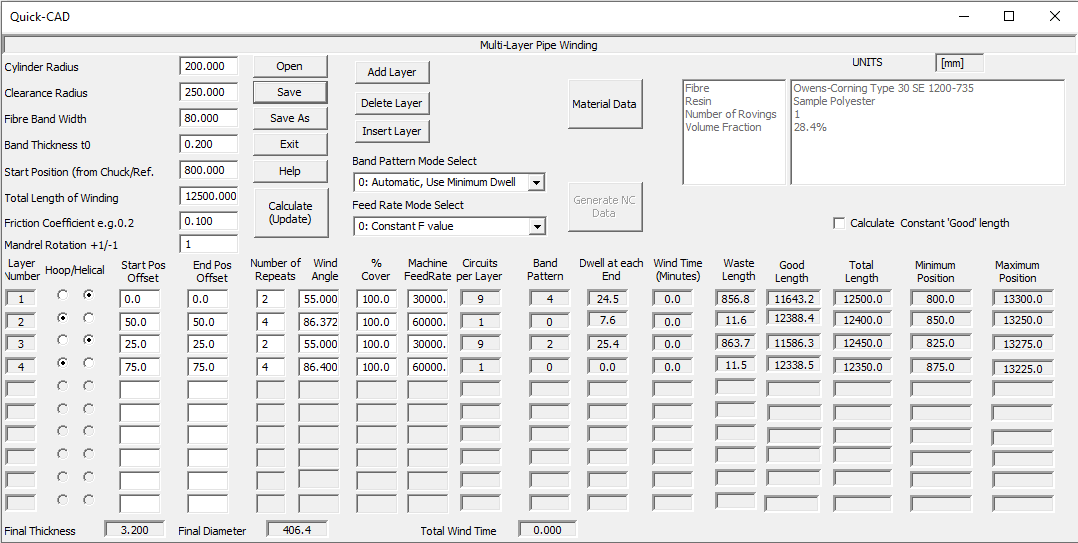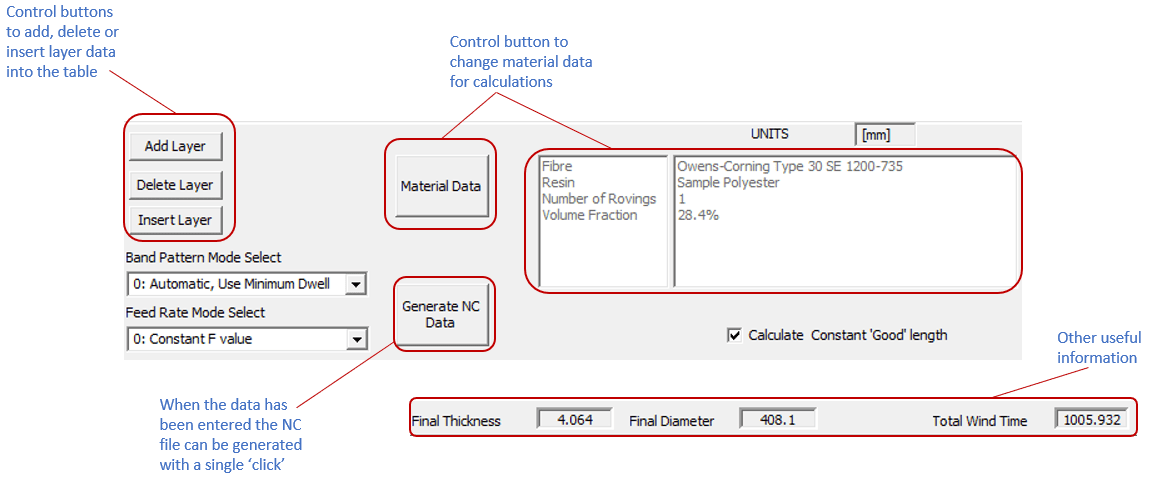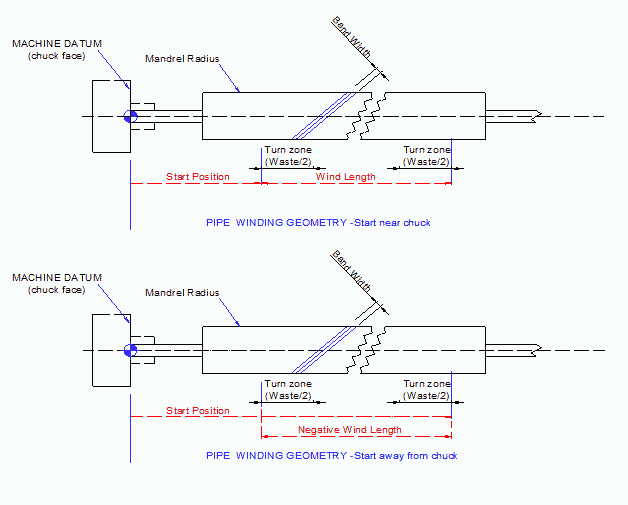# The Cadfil Pipewinder (QuickCad - Multi-Pipe Option)

## Pipe-Winder Overview

The Cadfil Pipe winding software is designed for higher angle winding (40-90 degrees) on pipes and is ideal for use on 2 axis winders. When Pipe-Winder is included as part of a higher specification package(e.g. Cadfil-Lite) then more machine axes may be available. Multi-layer and angle winding patterns can be quickly generated with both hoop (90 degree) and helical winding. Pipe winding is not suitable for low angles (less than 30 degrees) as this involves winding over an end-cap. For such applications Cadfil-Lite is more suitable. The thickness of the winding and winding times are automatically calculated. Each layer is calculated making allowance for the thickness of the previous winding to ensure band pattern and fibre angles are always optimum. For each later the turning length at the end where with winding angle is changing for the turning around is automatically calculated. For some applications this length is considered as waste as it is cut away after winding. As of Version 9.09 there are additional columns that give the 'good length' i.e. the part of the layer that is, at the angle that you asked for, as well as the axial positions of the ends of the layer are given. If the new "Calculate constant good length" box is ticked Cadfil finds the layer that has the longest turn (waste) length and then using the total wind length from the header data calculates the 'good' length. Then for all the other layers the length becomes the turn length plus the good length. In this way material is saved if the ends of the finished tube are to be cut away. the result of using two layer sample data in the dialog above is shown in the picture below.In the picture above in first green layer is followed by an automatically created joining path and then a single, much short (blue) hoop layer.

This software is driven from a single dialog screen. As of Version 9.09, it has the feature to minimise waste by automatically reducing the lengths of layers that have shorter turn-around lengths.
The image below is from Cadfil V9.51, optional length offsets are added. These can be used to adjust the length of a layer from the general length set so if values of 100mm and 200mm were set for a layers the pipe layer length will be reduced by 100m a the first end and 200mm at the second end. The offset can be negative. Such adjustments are often made to reduce the large 'dog bone' build up of thickness at the end of the wind.## Creating a program

Creating a program with pipe-winder is simple. There is a single window dialog box (data entry screen) show on the first page of this document. The steps for program generation are:

1) Enter the Header Information. Basic information such as mandrel diameter and the fibre band width that is constant for each layer.

2) Click The Add layer button to activate a new layer. Enter the data specific to that layer such as winding angle etc.

3) Click 'Add layer' to add the next layer. Layers can be deleted using the Delete layer button or a new layer can in inserted part way though the sequence using the Insert Layer button. In both these cases the layer number in the left most column is used to select where to insert or delete a layer. The date entry form allows for 10 separate layers. If more are required a second pipe winding can me made using the initial 'mandrel radius' as the final mandrel radius after the first 10 layers.

4) Click the 'Calculate(update)' button to fill out calculated values in the data table. You will be asked to save the data and must give a file name. Error messages will be given if data is incorrect or missing. You must correct the data.

5) After a successful Calculate (update)the Generate NC-Data button becomes active, click this to generate the complete winding program, after NC generate the wind times are added to the table. You get an option to view the program in the default text editor (normally notepad). The process creates a number of data files, the parameters entered are saved in a .par file. For each layer we automatically create a payout path(.pay) and mandrel (.mnd) file. If any joining paths are needed .pay and .mnd files are created for these also. Finally a combined winding file (.ctl) is created for the post-processor. For the parameters shown in the example earlier in this topic the .ctl file is shown below opened in the combine programs dialog. The parameter file was given the name "site_example.par", all the other files are created automatically. Note that an advanced user could take this .ctl file and add of program elements created by the "Pipe", "Multi-Hoop" or "Pipe-Joining" parametric options.## The Multi-Pipe 'header' data

A description of the 'header' data is shown in the following figureCylinder radius. Is the radius of the mandrel at the start of winding. If the mandrel diameter is 200mm the radius is 100mm.

Clearance Radius is the distance of the payout head (fibre comb or similar) from the mandrel rotation centre, so if the mandrel radius was 100mm and the clearance radius was 150mm the machine payout head will be 50mm (150-100) from the surface of the mandrel. For machines with more than 2 axis this position can be set automatically in the NC code. For 2 axis machine this must be set manually or a value that is possible (or fixed) on the machine must be entered. If the machine is a large distance from the mandrel accuracy will reduce (variation in wind length and band structure). For large mandrels (more than 1m diameters) a surface clearance of 250mm (10 inches) is OK. For small mandrels (<300mm diameter) a surface clearance of 50-75 mm (2-3 inches) is OK.

Fibre band-width is the true width of the fibres band as it lays onto the mandrel, if you considered it as a tape.

The Band thickness is the thickness of the fibre band as it lays down on the mandrel if you consider it as a tape. One way to measure is make a single layer of hoop and the band thickness is half the increase in mandrel diameter.

Start position. This is the position along the machine carriage where the winding will start. It is measured from a fixed position often the machine chuck face or headstock. This reference is set up in the post-processor configuration file. Your machine supplier or software supplier should be able to advice/or set up this file.

The total length of winding is exactly what it says. This included any turning length so if you need a length of tube with an exact wind angle all the way along it you will need to use a longer wind length to allow for the turning length. The waste is automatically calculated. So if you want say 2000mm at 45 degrees enter this data and 'calculate'. If the waste length is given as 200mm then increase the total length of winding to 2200mm. If a negative length is used the winding will start in the negative direction of the carriage, so the start position must be specified to suit this, there is a diagram later in this document. If the new "Calculate constant good length" box is ticked Cadfil finds the layer that has the longest turn (waste) length and then using the total wind length from the header data calculates the 'good' length. Then for all the other layers the length becomes the turn length plus the good length.

The friction coefficient is used to calculate the turning area. Cadfil supplies a default value but this can be changed by the user. If friction is increased the tuning lengths get shorter but if too high a value is used the fibres will slip. Typically a value of 0.1-0.2 is used.

Mandrel rotation +1/-1 is the direction the mandrel rotates, a value of +1 (default) give positive(+) mandrel rotation, -1 gives negative (-) mandrel rotation.## Layer Data Explained

The previous picture shows the data layout for each layers. New layers can be made using the add or insert buttons or deleted with the delete layer button. The data items are described below.

Hoop/Helical Buttons. Each layer is either hoop or helical. If hoop is selected the wind angle will be calculated to give a helical lead of one band with. If you wish the Bands to overlap or gap for a hoop winding use the 'cover factor' parameter. Helical winding creates a +/- layer at the wind angle specified by the user.

Number of repeats allow the layer to be repeated a number of times. For example with a helical winding setting 3 gives 3 +/- layers. It is not good to set the number of repeats to high numbers as the mandrel radius increases with each layer and the wind angle will thus change slightly with each repeat layer. Eventually gaps will start to appear between the bands. This effect is more pronounced if the mandrel has a small diameter as the change in thickness with each layer will be proportionately more. For hoop winding each layer is a single traverse., if an odd number is specified i.e. 1,3,5 the next layer will start at the other end of the winding length.

Wind angle is the helical angle in degrees, 0 is axial 90 is circumferential (hoop). For simple pipe winding (no end caps) angles below 40 degrees are not advisable as the turning length (waste) increased dramatically.

Cover factor is 100% by default but the user can over-ride it. This can be used to 'adjust' the band width. If a cover factor of 200% is used the programmed bandwidth will halve so the bands will overlap. For a cover factor of 50% the winding will have gaps. During winding the bandwidth may be slightly different for different wind angles so the user can adjust for this here if required.

Machine feed rate is the winding speed for each layer. The default value is from the machine configuration file. The winding machine supplier should assist in the setup of this file.

Circuits per layer, is a calculated value of how many circuit the winding program makes to complete one full layer. For hoop winding this is always 1.

Band pattern Number is the number of 'starts' and is explained on following pages of this document. There is no pattern as such for hoop winding.

Dwell rotation is additional mandrel rotation at the ends to make a particular band pattern work correctly. The amount of dwell can be minimised by keeping the default band pattern selection mode (mode 0).

Wind time is the layer wind time, a total is shown at the bottom. Times are calculated at NC generate stage. Waste length is the length out of the total wind length used for turning, where the wind angle is not true. Half of this amount will be at each end.

The Pull down menu for band pattern and feed rate mode selection are shown below. There are three options for band pattern, the user can choose that a pattern of 1  is automatically selected for each layer or the pattern with the smallest dwell is selected. In manual mode the user selects from a pattern table for each layer.## Wind The NC data - Start position explainedThe start position can be at either end of the mandrel.
To start away from the chuck and then initially towards it, use a negative Total Length of Winding (wind length in the lower diagram)
For hoop winding, each repeat (layer) is one traverse of the carriage. If the repeats are an odd number, then the next helical layer will automatically swap ends of the mandrel to start. This ensures that the winding is always continuous.

Updated: 11 September 2020